# How to Sum Range Ignoring Errors in Excel

This post will guide you how to sum range of cells ignoring Errors in Excel. How do I sum and ignore errors with a formula in Excel. How to sum range ignoring all error values with VBA macro in Excel.

## Sum Range Ignoring Errors with Formula

Assuming that you have a list of data in range A1:C4, in which contain numeric values and the error values, such as: #NAME?, #DIV/0, etc. and you want to sum this range but ignoring all error values. How to do it. You can use an Excel Array formula based on the SUM function, the IF function and the ISERROR function to achieve the result. Like this:

`=SUM(IF(ISERROR(A1:C4),””,A1:C4))`

You need to type this formula into a blank cell and press Ctrl + Shift + Enter keys on your keyboard to change this formula as array formula.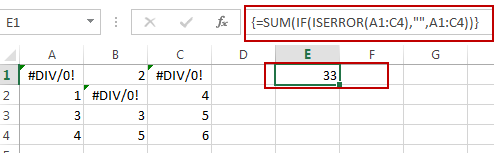## Sum Range Ignoring Errors with VBA

You can also use an Excel VBA Macro to achieve the same result of summing a selected range of cells ignoring all error values. Here are the steps:

#1 open your excel workbook and then click on “Visual Basic” command under DEVELOPER Tab, or just press “ALT+F11” shortcut.#2 then the “Visual Basic Editor” window will appear.

#3 click “Insert” ->”Module” to create a new module.#4 paste the below VBA code  into the code window. Then clicking “Save” button.

```Sub SumRangeIgnoringError()

Set myRange = Application.Selection
Set myRange = Application.InputBox("Select one Range:", "SumRangeIgnoringError", myRange.Address, Type:=8)
For Each myCell In myRange
If Not (IsError(myCell.Value)) Then
lSum = lSum + myCell.Value
End If
Next
MsgBox lSum, , "SumRangeIgnoringError"
End Sub```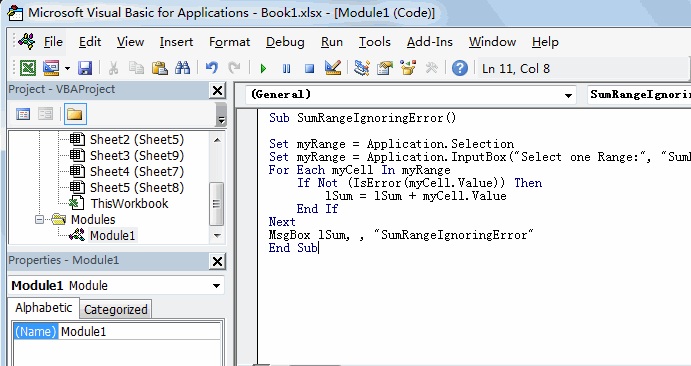#5 back to the current worksheet, then run the above excel macro. Click Run button.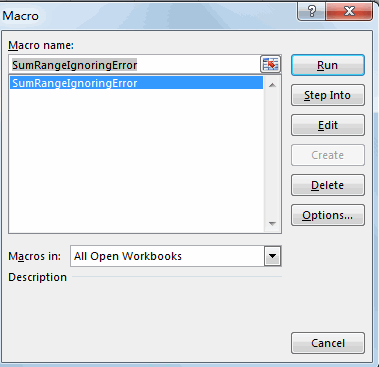#6 Please select one range that contain error values. Click OK button.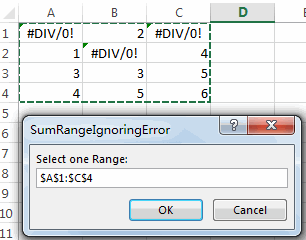#7 Let’s see the result: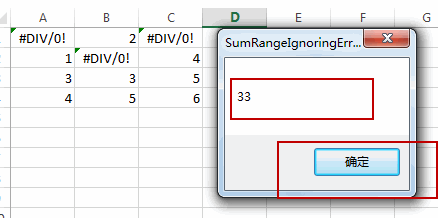### Related Functions

• Excel SUM function
The Excel SUM function will adds all numbers in a range of cells and returns the sum of these values. You can add individual values, cell references or ranges in excel.The syntax of the SUM function is as below:= SUM(number1,[number2],…)…
• Excel IF function
The Excel IF function perform a logical test to return one value if the condition is TRUE and return another value if the condition is FALSE. The IF function is a build-in function in Microsoft Excel and it is categorized as a Logical Function.The syntax of the IF function is as below:= IF (condition, [true_value], [false_value])….
• Excel ISERROR function
The Excel ISERROR function used to check for any error type that excel generates and it returns TRUE for any error type, and the ISERR function also can be checked for error values except #N/A error, it returns TRUE while the error is #N/A. The syntax of the ISERROR function is as below:= ISERROR (value)….